# NCERT Exemplar Class 12 Chemistry Solutions for Chapter 6 - General Principles and Processes of Isolation of Elements

NCERT Class 12 Chemistry Exemplar Solutions for Chapter 6 General Principles and Processes of Isolation of Elements are significant resource materials that students require to prepare for their CBSE Class 12 and graduate entrance examinations. The NCERT Class 12 Chemistry Exemplar for Chapter 6 has solutions to exemplar questions and problems in the NCERT exemplar book, along with extra questions prepared by BYJU’S Chemistry teachers. The NCERT Class 12 Chemistry Exemplar for Chapter 6 PDF has MCQs, short answer questions, match the following, fill in the blanks, true or false, numerical questions, worksheets and exercises to assist Class 12 Science students in preparing well.

## Download the PDF of the NCERT Exemplar for Class 12 Chemistry Chapter 6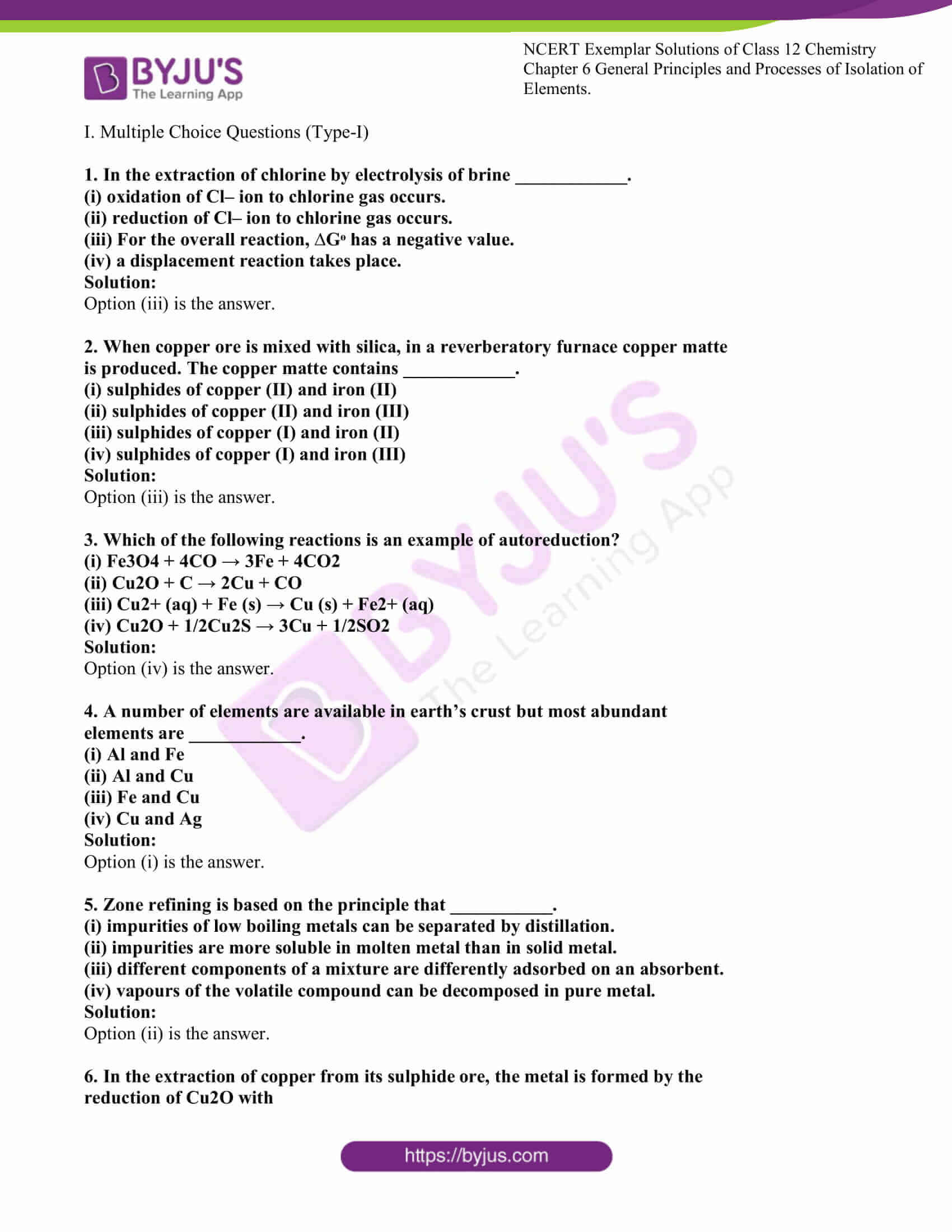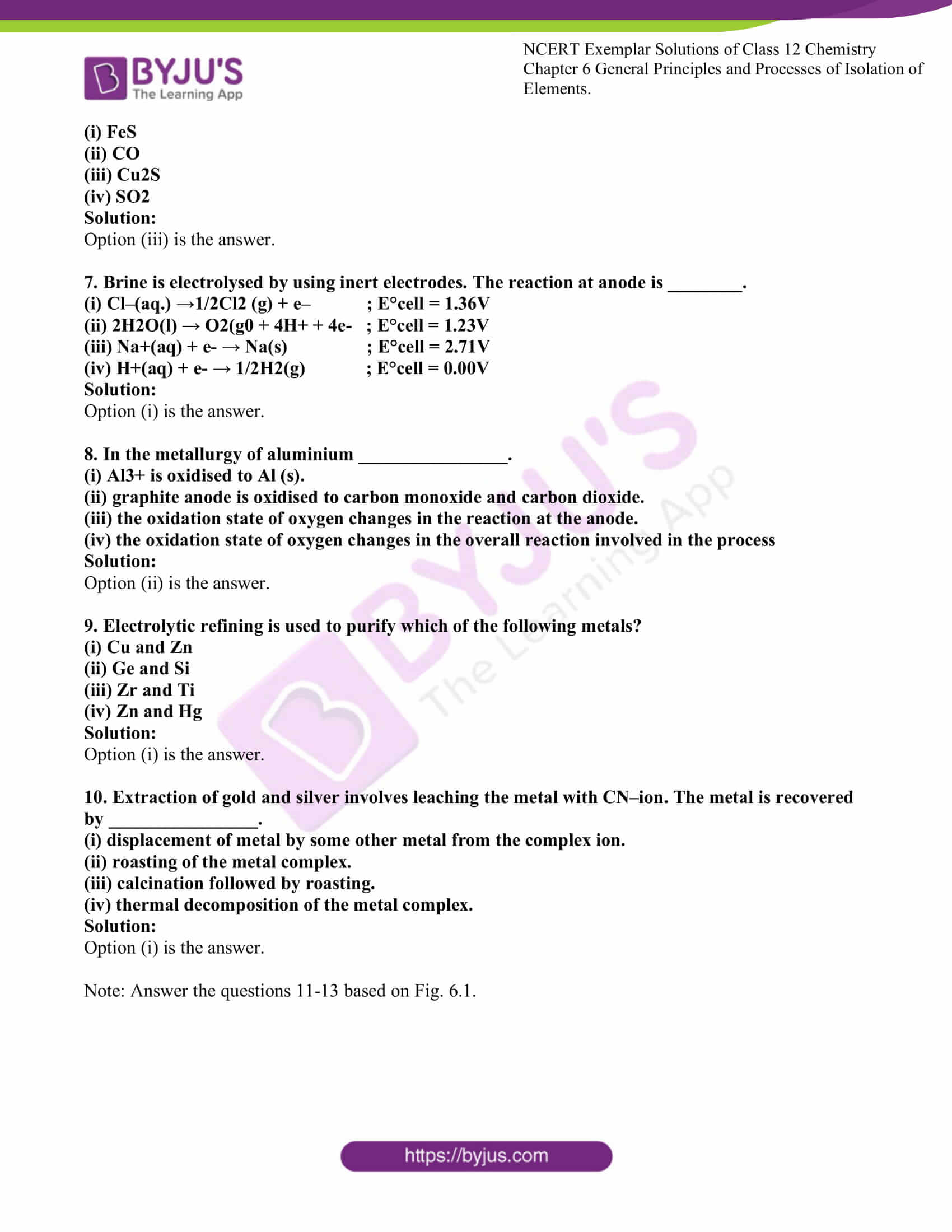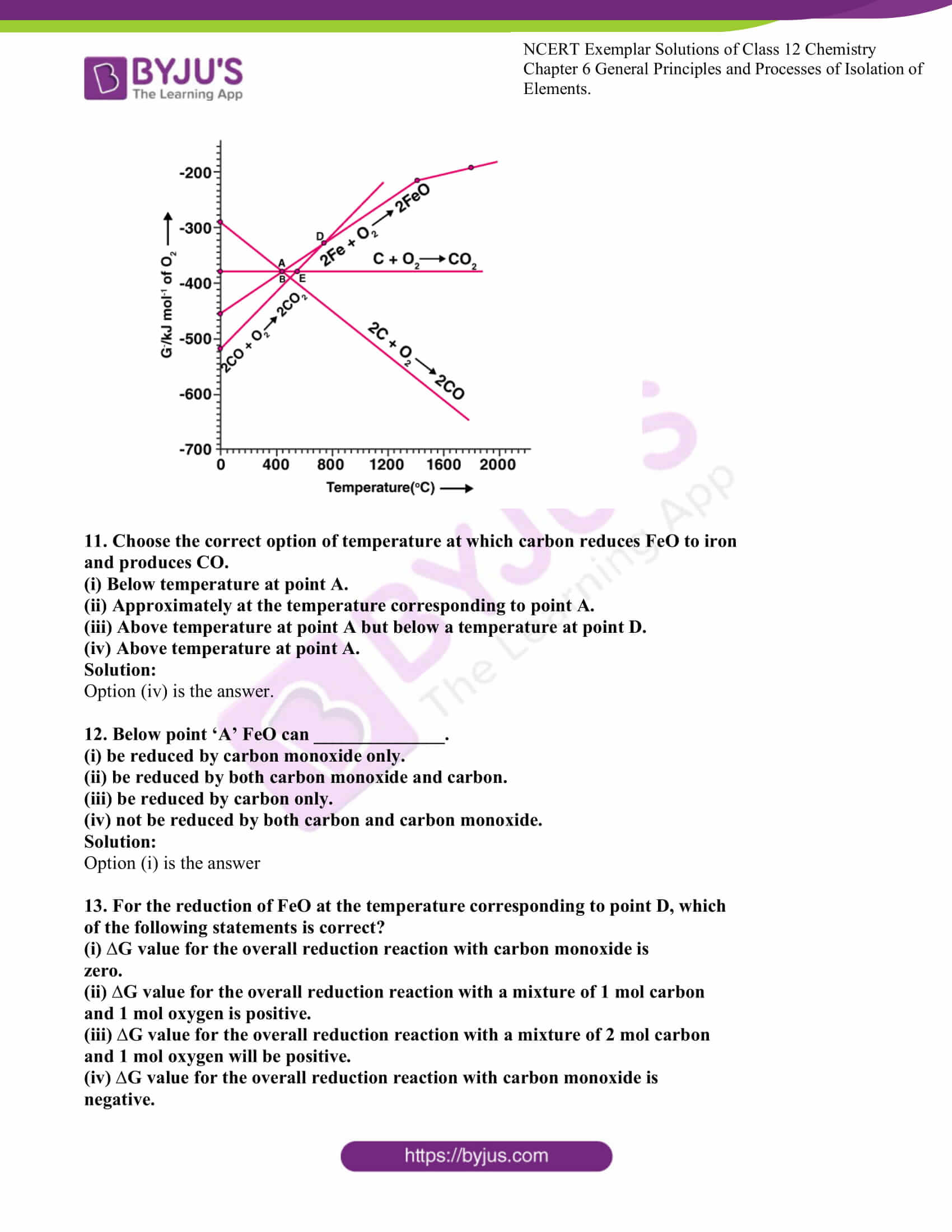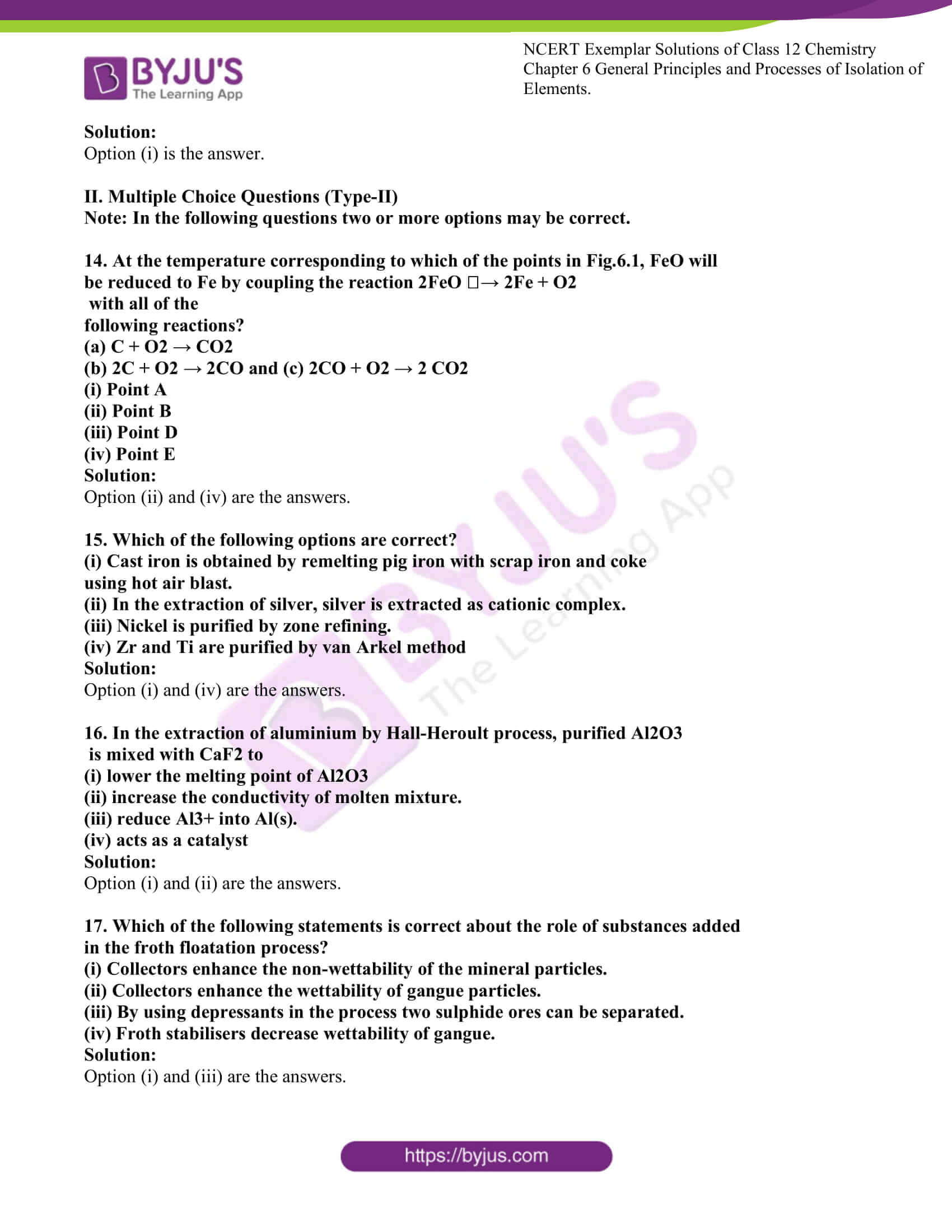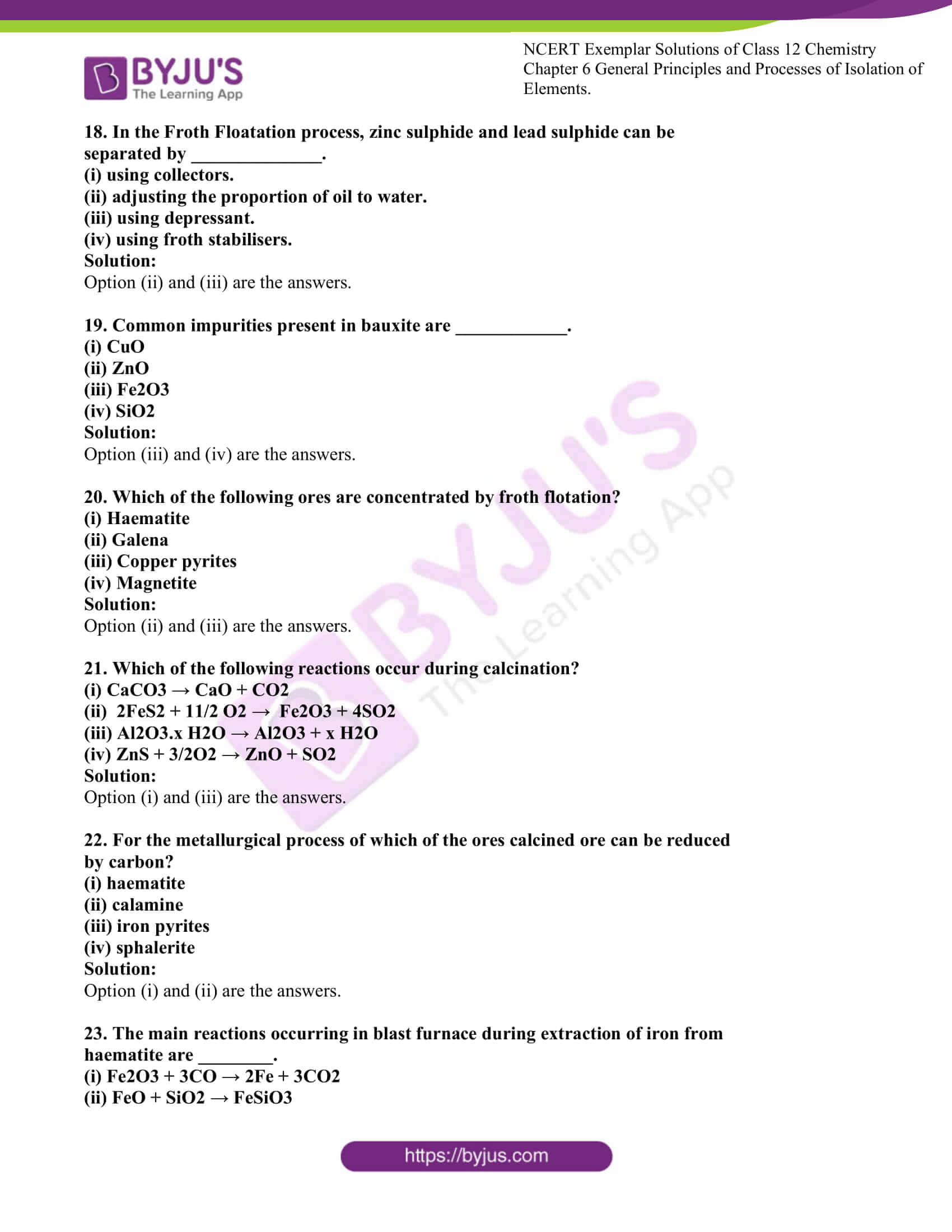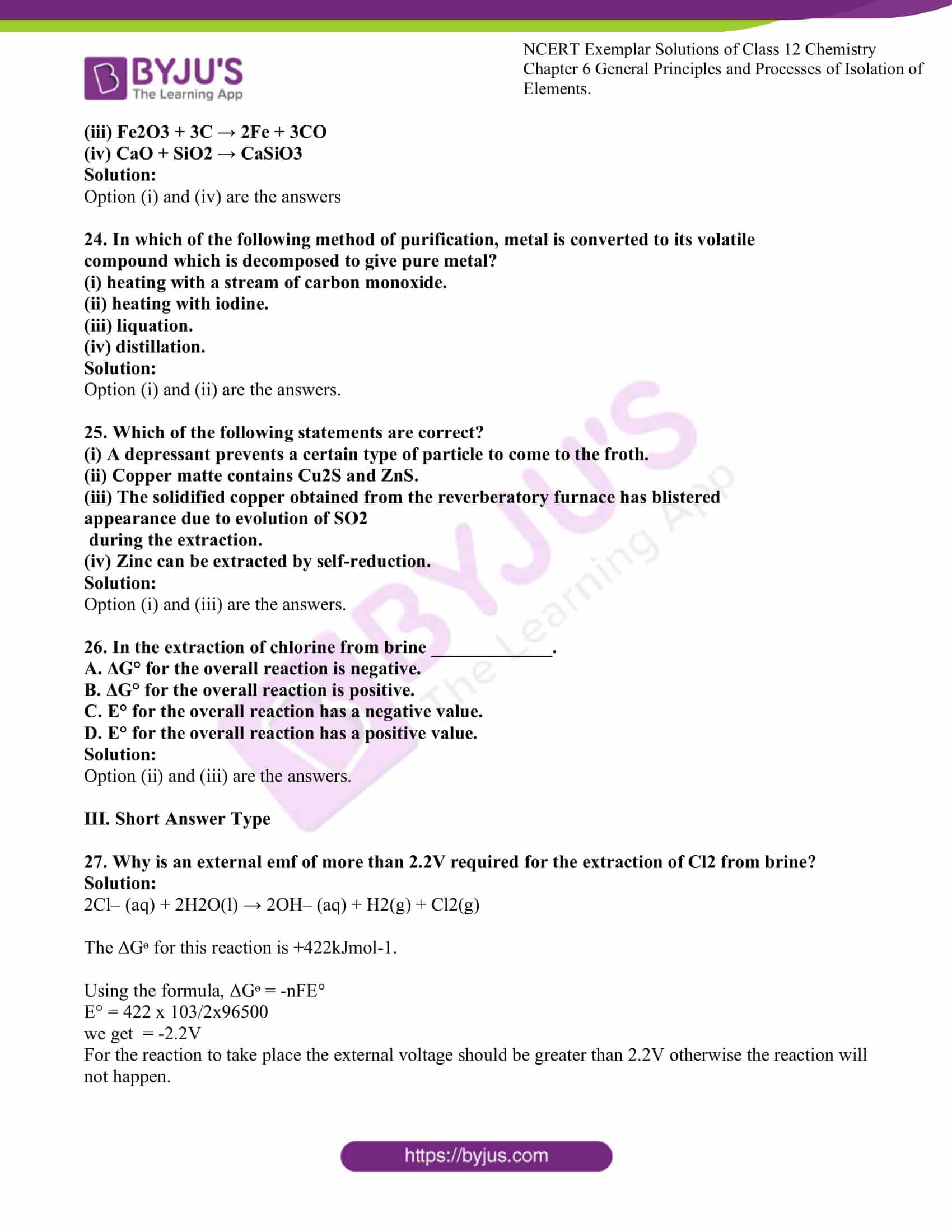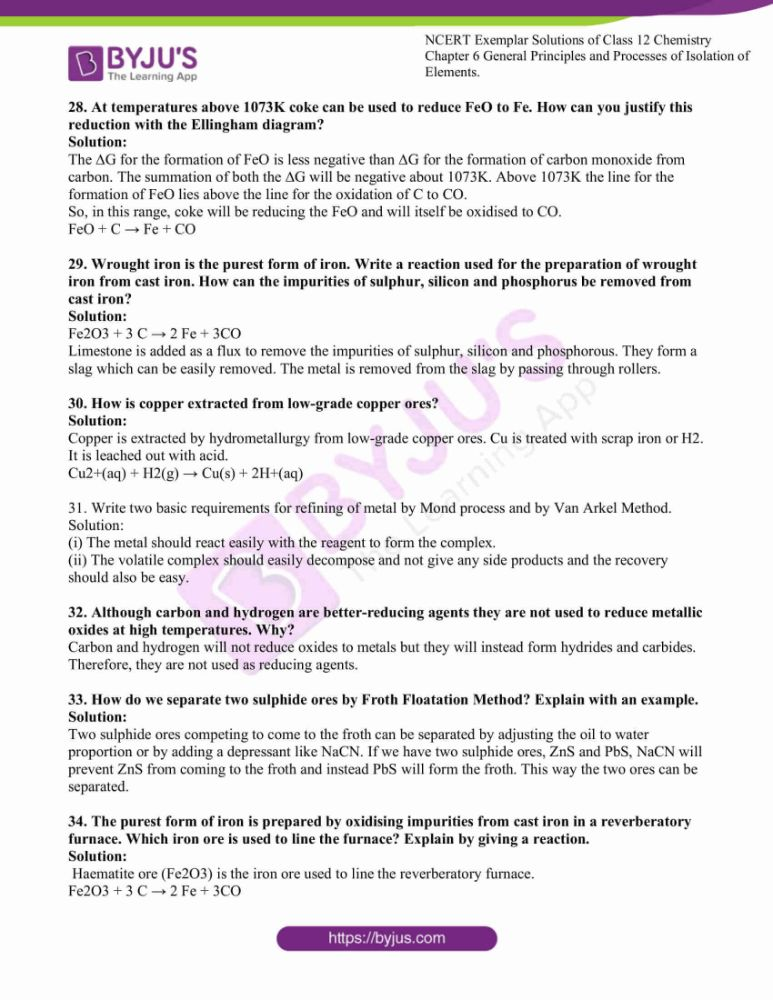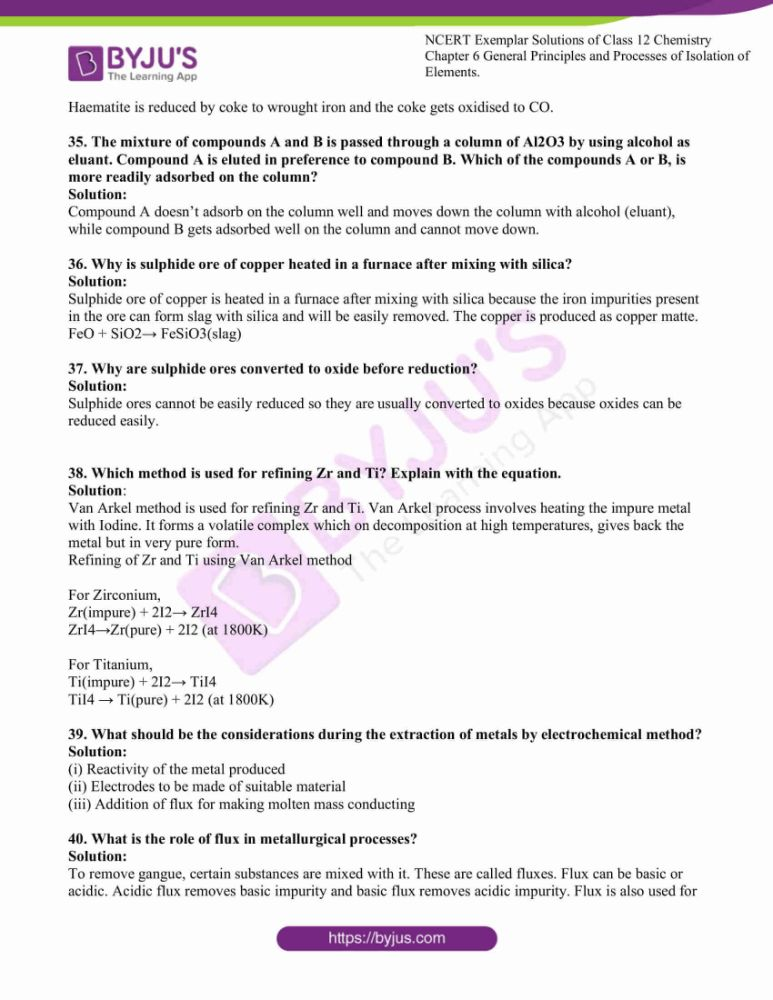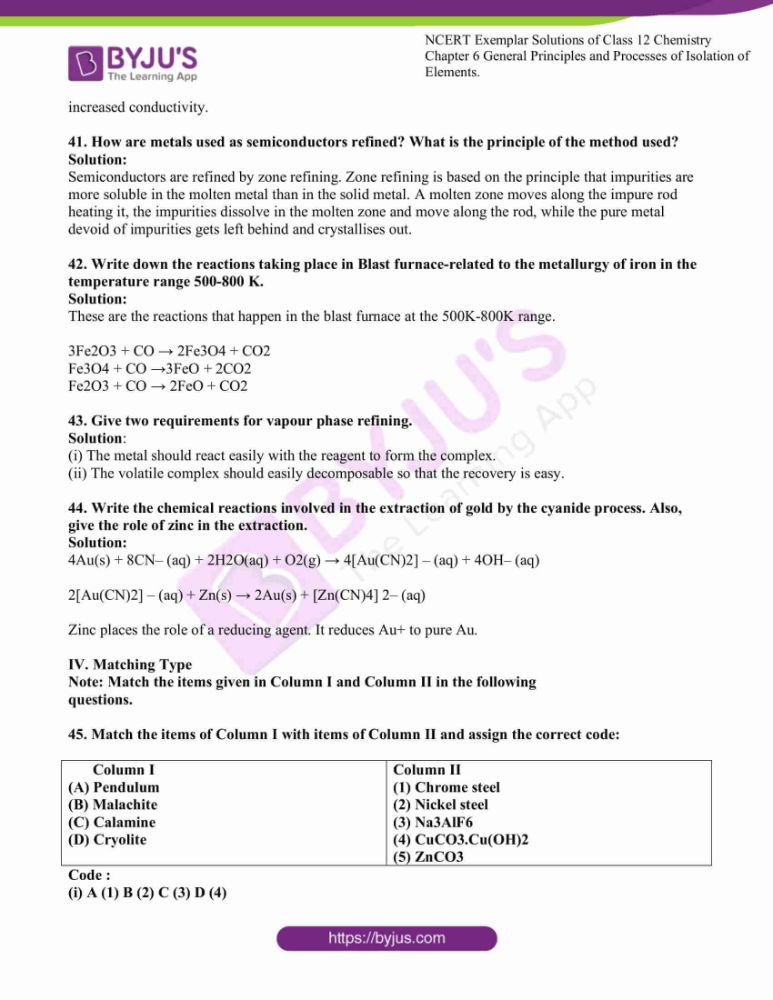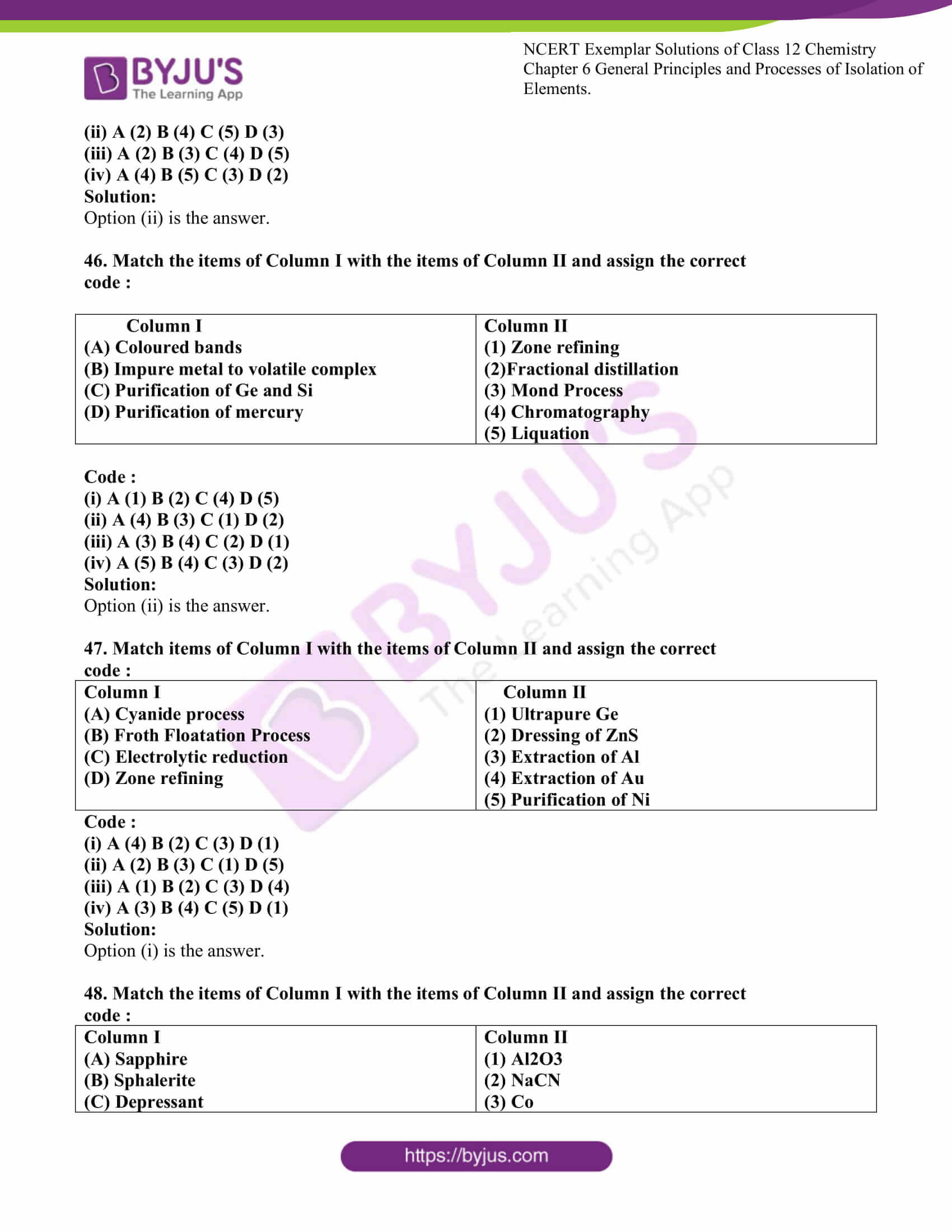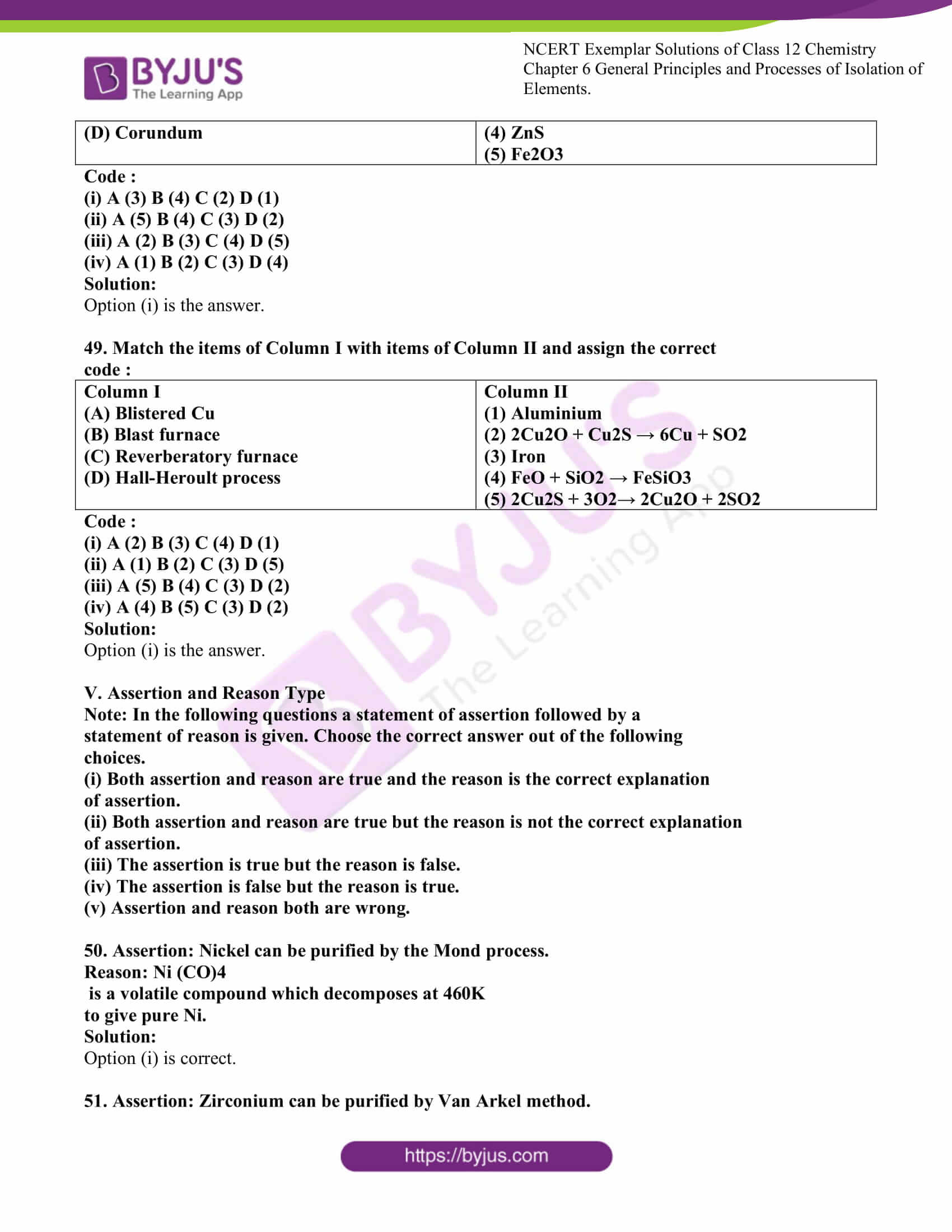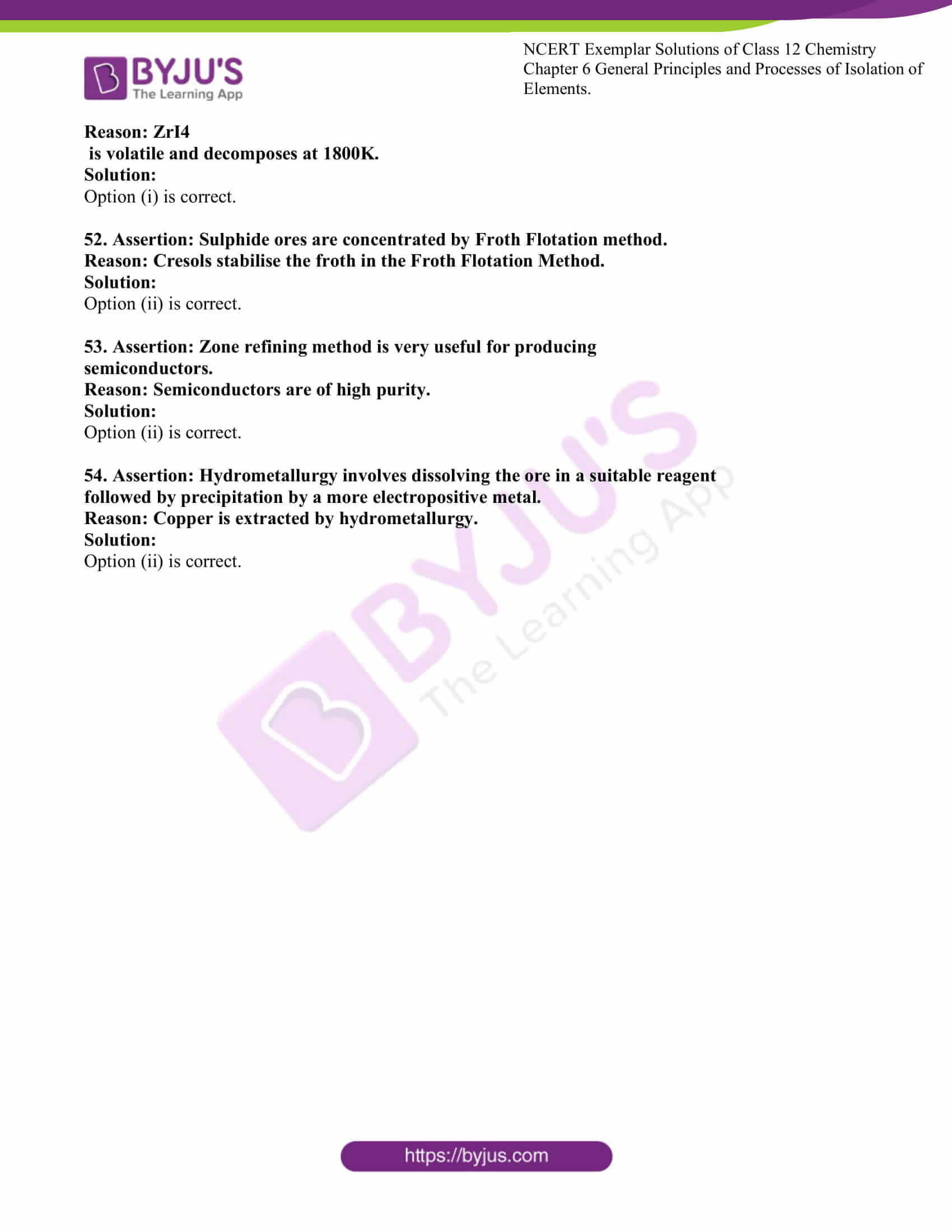### Access Solutions to the NCERT Exemplar Class 12 Chemistry Chapter 6

I. Multiple Choice Questions (Type-I)

1. In the extraction of chlorine by electrolysis of brine ____________.

(i) oxidation of Cl– ion to chlorine gas occurs.

(ii) reduction of Cl– ion to chlorine gas occurs.

(iii) For the overall reaction, ∆Gᶱ has a negative value.

(iv) a displacement reaction takes place.

Solution:

2. When copper ore is mixed with silica, in a reverberatory furnace copper matte

is produced. The copper matte contains ____________.

(i) sulphides of copper (II) and iron (II)

(ii) sulphides of copper (II) and iron (III)

(iii) sulphides of copper (I) and iron (II)

(iv) sulphides of copper (I) and iron (III)Solution:

3. Which of the following reactions is an example of autoreduction?

(i) Fe3O4 + 4CO → 3Fe + 4CO2

(ii) Cu2O + C → 2Cu + CO

(iii) Cu2+ (aq) + Fe (s) → Cu (s) + Fe2+ (aq)

(iv) Cu2O + 1/2Cu2S → 3Cu + 1/2SO2

Solution:

4. A number of elements are available in earth’s crust but most abundant

elements are ____________.

(i) Al and Fe

(ii) Al and Cu

(iii) Fe and Cu

(iv) Cu and Ag

Solution:

5. Zone refining is based on the principle that ___________.

(i) impurities of low boiling metals can be separated by distillation.

(ii) impurities are more soluble in molten metal than in solid metal.

(iii) different components of a mixture are differently adsorbed on an absorbent.

(iv) vapours of the volatile compound can be decomposed in pure metal.

Solution:

6. In the extraction of copper from its sulphide ore, the metal is formed by the

reduction of Cu2O with

(i) FeS

(ii) CO

(iii) Cu2S

(iv) SO2

Solution:

7. Brine is electrolysed by using inert electrodes. The reaction at anode is ________.

(i) Cl–(aq.) →1/2Cl2 (g) + e– ; E°cell = 1.36V

(ii) 2H2O(l) → O2(g0 + 4H+ + 4e- ; E°cell = 1.23V

(iii) Na+(aq) + e- → Na(s) ; E°cell = 2.71V

(iv) H+(aq) + e- → 1/2H2(g) ; E°cell = 0.00V

Solution:

8. In the metallurgy of aluminium ________________.

(i) Al3+ is oxidised to Al (s).

(ii) graphite anode is oxidised to carbon monoxide and carbon dioxide.

(iii) the oxidation state of oxygen changes in the reaction at the anode.

(iv) the oxidation state of oxygen changes in the overall reaction involved in the process

Solution:

9. Electrolytic refining is used to purify which of the following metals?

(i) Cu and Zn

(ii) Ge and Si

(iii) Zr and Ti

(iv) Zn and Hg

Solution:

10. Extraction of gold and silver involves leaching the metal with CN–ion. The metal is recovered by ________________.

(i) displacement of metal by some other metal from the complex ion.

(ii) roasting of the metal complex.

(iii) calcination followed by roasting.

(iv) thermal decomposition of the metal complex.

Solution:

Note: Answer the questions 11-13 based on Fig. 6.1.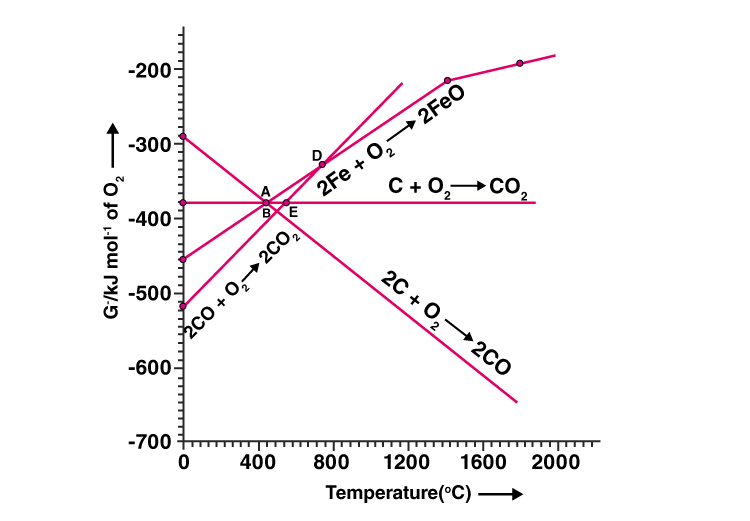11. Choose the correct option of temperature at which carbon reduces FeO to iron

and produces CO.

(i) Below temperature at point A.

(ii) Approximately at the temperature corresponding to point A.

(iii) Above temperature at point A but below a temperature at point D.

(iv) Above temperature at point A.

Solution:

12. Below point ‘A’ FeO can ______________.

(i) be reduced by carbon monoxide only.

(ii) be reduced by both carbon monoxide and carbon.

(iii) be reduced by carbon only.

(iv) not be reduced by both carbon and carbon monoxide.

Solution:

13. For the reduction of FeO at the temperature corresponding to point D, which

of the following statements is correct?

(i) ∆G value for the overall reduction reaction with carbon monoxide is

zero.

(ii) ∆G value for the overall reduction reaction with a mixture of 1 mol carbon

and 1 mol oxygen is positive.

(iii) ∆G value for the overall reduction reaction with a mixture of 2 mol carbon

and 1 mol oxygen will be positive.

(iv) ∆G value for the overall reduction reaction with carbon monoxide is

negative.

Solution:

II. Multiple Choice Questions (Type-II)

Note: In the following questions two or more options may be correct.

14. At the temperature corresponding to which of the points in Fig.6.1, FeO will

be reduced to Fe by coupling the reaction 2FeO → 2Fe + O2

with all of the

following reactions?

(a) C + O2 → CO2

(b) 2C + O2 → 2CO and (c) 2CO + O2 → 2 CO2

(i) Point A

(ii) Point B

(iii) Point D

(iv) Point ESolution:

Option (ii) and (iv) are the answers.

15. Which of the following option are correct?

(i) Cast iron is obtained by remelting pig iron with scrap iron and coke

using hot air blast.

(ii) In the extraction of silver, silver is extracted as cationic complex.

(iii) Nickel is purified by zone refining.

(iv) Zr and Ti are purified by van Arkel method

Solution:

Option (i) and (iv) are the answers.

16. In the extraction of aluminium by Hall-Heroult process, purified Al2O3

is mixed with CaF2 to

(i) lower the melting point of Al2O3

(ii) increase the conductivity of molten mixture.

(iii) reduce Al3+ into Al(s).

(iv) acts as a catalyst

Solution:

Option (i) and (ii) are the answers.

17. Which of the following statements is correct about the role of substances added

in the froth floatation process?

(i) Collectors enhance the non-wettability of the mineral particles.

(ii) Collectors enhance the wettability of gangue particles.

(iii) By using depressants in the process two sulphide ores can be separated.

(iv) Froth stabilisers decrease wettability of gangue.

Solution:

Option (i) and (iii) are the answers.

18. In the Froth Floatation process, zinc sulphide and lead sulphide can be

separated by ______________.

(i) using collectors.

(ii) adjusting the proportion of oil to water.

(iii) using depressant.

(iv) using froth stabilisers.

Solution:

Option (ii) and (iii) are the answers.

19. Common impurities present in bauxite are ____________.

(i) CuO

(ii) ZnO

(iii) Fe2O3

(iv) SiO2

Solution:

Option (iii) and (iv) are the answers.

20. Which of the following ores are concentrated by froth flotation?

(i) Haematite

(ii) Galena

(iii) Copper pyrites

(iv) MagnetiteSolution:

Option (ii) and (iii) are the answers.

21. Which of the following reactions occur during calcination?

(i) CaCO3 → CaO + CO2

(ii) 2FeS2 + 11/2 O2 → Fe2O3 + 4SO2

(iii) Al2O3.x H2O → Al2O3 + x H2O

(iv) ZnS + 3/2O2 → ZnO + SO2

Solution:

Option (i) and (iii) are the answers.

22. For the metallurgical process of which of the ores calcined ore can be reduced

by carbon?

(i) haematite

(ii) calamine

(iii) iron pyrites

(iv) sphalerite

Solution:

Option (i) and (ii) are the answers.

23. The main reactions occurring in the blast furnace during the extraction of iron from

haematite are ________.

(i) Fe2O3 + 3CO → 2Fe + 3CO2

(ii) FeO + SiO2 → FeSiO3

(iii) Fe2O3 + 3C → 2Fe + 3CO

(iv) CaO + SiO2 → CaSiO3

Solution:

Option (i) and (iv) are the answers

24. In which of the following method of purification, metal is converted to its volatile

compound which is decomposed to give pure metal?

(i) heating with a stream of carbon monoxide.

(ii) heating with iodine.

(iii) liquation.

(iv) distillation.

Solution:

Option (i) and (ii) are the answers.

25. Which of the following statements are correct?

(i) A depressant prevents a certain type of particle to come to the froth.

(ii) Copper matte contains Cu2S and ZnS.

(iii) The solidified copper obtained from the reverberatory furnace has blistered

appearance due to evolution of SO2

during the extraction.

(iv) Zinc can be extracted by self-reduction.

Solution:

Option (i) and (iii) are the answers.

26. In the extraction of chlorine from brine _____________.

A. ΔG° for the overall reaction is negative.

B. ΔG° for the overall reaction is positive.

C. E° for the overall reaction has a negative value.

D. E° for the overall reaction has a positive value.

Solution:

Option (ii) and (iii) are the answers.

27. Why is an external emf of more than 2.2V required for the extraction of Cl2 from brine?

Solution:

2Cl– (aq) + 2H2O(l) → 2OH– (aq) + H2(g) + Cl2(g)

The ΔGᶱ for this reaction is +422kJmol-1.

Using the formula, ΔGᶱ = -nFE°

E° = 422 x 103/2×96500

we get = -2.2V

For the reaction to take place the external voltage should be greater than 2.2V otherwise the reaction will not happen.

28. At temperatures above 1073K coke can be used to reduce FeO to Fe. How can you justify this reduction with the Ellingham diagram?

Solution:

The ∆G for the formation of FeO is less negative than ∆G for the formation of carbon monoxide from carbon. The summation of both the ∆G will be negative about 1073K. Above 1073K the line for the formation of FeO lies above the line for the oxidation of C to CO.

So, in this range, coke will be reducing the FeO and will itself be oxidised to CO.

FeO + C → Fe + CO

29. Wrought iron is the purest form of iron. Write a reaction used for the preparation of wrought iron from cast iron. How can the impurities of sulphur, silicon and phosphorus be removed from cast iron?

Solution:

Fe2O3 + 3 C → 2 Fe + 3CO

Limestone is added as a flux to remove the impurities of sulphur, silicon and phosphorous. They form a slag which can be easily removed. The metal is removed from the slag by passing through rollers.

30. How is copper extracted from low-grade copper ores?

Solution:

Copper is extracted by hydrometallurgy from low-grade copper ores. Cu is treated with scrap iron or H2. It is leached out with acid.

Cu2+(aq) + H2(g) → Cu(s) + 2H+(aq)

31. Write two basic requirements for refining of metal by Mond process and by Van Arkel Method.

Solution:

(i) The metal should react easily with the reagent to form the complex.

(ii) The volatile complex should easily decompose and not give any side products and the recovery should also be easy.

32. Although carbon and hydrogen are better-reducing agents they are not used to reduce metallic oxides at high temperatures. Why?

Carbon and hydrogen will not reduce oxides to metals but they will instead form hydrides and carbides. Therefore, they are not used as reducing agents.

33. How do we separate two sulphide ores by Froth Floatation Method? Explain with an example.

Solution:

Two sulphide ores competing to come to the froth can be separated by adjusting the oil to water proportion or by adding a depressant like NaCN. If we have two sulphide ores, ZnS and PbS, NaCN will prevent ZnS from coming to the froth and instead PbS will form the froth. This way the two ores can be separated.

34. The purest form of iron is prepared by oxidising impurities from cast iron in a reverberatory furnace. Which iron ore is used to line the furnace? Explain by giving a reaction.

Solution:

Haematite ore (Fe2O3) is the iron ore used to line the reverberatory furnace.

Fe2O3 + 3 C → 2 Fe + 3CO

Haematite is reduced by coke to wrought iron and the coke gets oxidised to CO.

35. The mixture of compounds A and B is passed through a column of Al2O3 by using alcohol as eluant. Compound A is eluted in preference to compound B. Which of the compounds A or B, is more readily adsorbed on the column?

Solution:

Compound A doesn’t adsorb on the column well and moves down the column with alcohol (eluant), while compound B gets adsorbed well on the column and cannot move down.

36. Why is sulphide ore of copper heated in a furnace after mixing with silica?

Solution:

Sulphide ore of copper is heated in a furnace after mixing with silica because the iron impurities present in the ore can form slag with silica and will be easily removed. The copper is produced as copper matte.

FeO + SiO2→ FeSiO3(slag)

37. Why are sulphide ores converted to oxide before reduction?

Solution:

Sulphide ores cannot be easily reduced so they are usually converted to oxides because oxides can be reduced easily.

38. Which method is used for refining Zr and Ti? Explain with the equation.

Solution:

Van Arkel method is used for refining Zr and Ti. Van Arkel process involves heating the impure metal with Iodine. It forms a volatile complex which on decomposition at high temperatures, gives back the metal but in very pure form.

Refining of Zr and Ti using Van Arkel method

For Zirconium,

Zr(impure) + 2I2→ ZrI4

ZrI4→Zr(pure) + 2I2 (at 1800K)

For Titanium,

Ti(impure) + 2I2→ TiI4

TiI4 → Ti(pure) + 2I2 (at 1800K)

39. What should be the considerations during the extraction of metals by electrochemical method?

Solution:

(i) Reactivity of the metal produced

(ii) Electrodes to be made of suitable material

(iii) Addition of flux for making molten mass conducting

40. What is the role of flux in metallurgical processes?

Solution:

To remove gangue, certain substances are mixed with it. These are called fluxes. Flux can be basic or acidic. Acidic flux removes basic impurity and basic flux removes acidic impurity. Flux is also used for increased conductivity.

41. How are metals used as semiconductors refined? What is the principle of the method used?

Solution:

Semiconductors are refined by zone refining. Zone refining is based on the principle that impurities are more soluble in the molten metal than in the solid metal. A molten zone moves along the impure rod heating it, the impurities dissolve in the molten zone and move along the rod, while the pure metal devoid of impurities gets left behind and crystallises out.

42. Write down the reactions taking place in Blast furnace-related to the metallurgy of iron in the temperature range 500-800 K.

Solution:

These are the reactions that happen in the blast furnace at the 500K-800K range.

3Fe2O3 + CO → 2Fe3O4 + CO2

Fe3O4 + CO →3FeO + 2CO2

Fe2O3 + CO → 2FeO + CO2

43. Give two requirements for vapour phase refining.

Solution:

(i) The metal should react easily with the reagent to form the complex.

(ii) The volatile complex should easily decomposable so that the recovery is easy.

44. Write the chemical reactions involved in the extraction of gold by the cyanide process. Also, give the role of zinc in the extraction.

Solution:

4Au(s) + 8CN– (aq) + 2H2O(aq) + O2(g) → 4[Au(CN)2] – (aq) + 4OH– (aq)

2[Au(CN)2] – (aq) + Zn(s) → 2Au(s) + [Zn(CN)4] 2– (aq)

Zinc places the role of a reducing agent. It reduces Au+ to pure Au.

IV. Matching Type

Note: Match the items given in Column I and Column II in the following

questions.

45. Match the items of Column I with items of Column II and assign the correct code:

 Column I (A) Pendulum (B) Malachite (C) Calamine (D) Cryolite Column II (1) Chrome steel (2) Nickel steel (3) Na3AlF6 (4) CuCO3.Cu(OH)2 (5) ZnCO3

Code :

(i) A (1) B (2) C (3) D (4)

(ii) A (2) B (4) C (5) D (3)

(iii) A (2) B (3) C (4) D (5)

(iv) A (4) B (5) C (3) D (2)

Solution:

46. Match the items of Column I with the items of Column II and assign the correct

code :

 Column I (A) Coloured bands (B) Impure metal to volatile complex (C) Purification of Ge and Si (D) Purification of mercury Column II (1) Zone refining (2)Fractional distillation (3) Mond Process (4) Chromatography (5) Liquation

Code :

(i) A (1) B (2) C (4) D (5)

(ii) A (4) B (3) C (1) D (2)

(iii) A (3) B (4) C (2) D (1)

(iv) A (5) B (4) C (3) D (2)

Solution:

47. Match items of Column I with the items of Column II and assign the correct

code :

 Column I (A) Cyanide process (B) Froth Floatation Process (C) Electrolytic reduction (D) Zone refining Column II (1) Ultrapure Ge (2) Dressing of ZnS (3) Extraction of Al (4) Extraction of Au (5) Purification of Ni

Code :

(i) A (4) B (2) C (3) D (1)

(ii) A (2) B (3) C (1) D (5)

(iii) A (1) B (2) C (3) D (4)

(iv) A (3) B (4) C (5) D (1)Solution:

48. Match the items of Column I with the items of Column II and assign the correct

code :

 Column I (A) Sapphire (B) Sphalerite (C) Depressant (D) Corundum Column II (1) Al2O3 (2) NaCN (3) Co (4) ZnS (5) Fe2O3

Code :

(i) A (3) B (4) C (2) D (1)

(ii) A (5) B (4) C (3) D (2)

(iii) A (2) B (3) C (4) D (5)

(iv) A (1) B (2) C (3) D (4)

Solution:

49. Match the items of Column I with items of Column II and assign the correct

code :

 Column I (A) Blistered Cu (B) Blast furnace (C) Reverberatory furnace (D) Hall-Heroult process Column II (1) Aluminium (2) 2Cu2O + Cu2S → 6Cu + SO2 (3) Iron (4) FeO + SiO2 → FeSiO3 (5) 2Cu2S + 3O2→ 2Cu2O + 2SO2

Code :

(i) A (2) B (3) C (4) D (1)

(ii) A (1) B (2) C (3) D (5)

(iii) A (5) B (4) C (3) D (2)

(iv) A (4) B (5) C (3) D (2)

Solution:

V. Assertion and Reason Type

Note: In the following questions a statement of assertion followed by a

statement of reason is given. Choose the correct answer out of the following

choices.

(i) Both assertion and reason are true and the reason is the correct explanation

of assertion.

(ii) Both assertion and reason are true but the reason is not the correct explanation

of assertion.

(iii) The assertion is true but the reason is false.

(iv) The assertion is false but the reason is true.

(v) Assertion and reason both are wrong.

50. Assertion: Nickel can be purified by the Mond process.

Reason: Ni (CO)4

is a volatile compound which decomposes at 460K

to give pure Ni.

Solution:

Option (i) is correct.

51. Assertion: Zirconium can be purified by Van Arkel method.

Reason: ZrI4

is volatile and decomposes at 1800K.Solution:

Option (i) is correct.

52. Assertion: Sulphide ores are concentrated by Froth Flotation method.

Reason: Cresols stabilise the froth in the Froth Flotation Method.

Solution:

Option (ii) is correct.

53. Assertion: Zone refining method is very useful for producing

semiconductors.

Reason: Semiconductors are of high purity.

Solution:

Option (ii) is correct.

54. Assertion: Hydrometallurgy involves dissolving the ore in a suitable reagent

followed by precipitation by a more electropositive metal.

Reason: Copper is extracted by hydrometallurgy.

Solution:

Option (ii) is correct.

### Class 12 Chemistry NCERT Exemplar for General Principles and Processes of Isolation of Elements

The chapter General Principles and Processes of Isolation of Elements teaches students about different terms like minerals, calcination, concentration, good deed, roasting, ores and refining. They also learn the principles of oxidation and reduction as applied to the extraction procedures.
The Important Concepts in Chapter 6 General Principles and Processes of Isolation of Elements

1. Occurrence of Metals
2. The Concentration of Ores: Hydraulic Washing, Magnetic Separation, Froth Flotation Method and Leaching
3. Extraction of Crude Metal from Concentrated Ore
4. Thermodynamic Principles of Metallurgy and Applications
5. Electrochemical Principles of Metallurgy, Oxidation-Reduction and Refining,
6. Uses of Aluminium, Copper, Zinc and Iron

The NCERT Exemplar Class 12 Chapter 6 General Principles and Processes of Isolation of Elements is provided here. Click the below link to download the PDF.

### Why Opt for BYJU’S

We, at BYJU’S, provide chapter-wise Class 12 Chemistry exemplar questions to help students with their studies. All the NCERT solutions are explained in simple language and format for them to learn effectively. These study materials are available in PDF format, and students can download them easily from BYJU’S website.